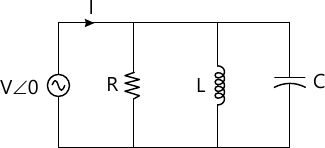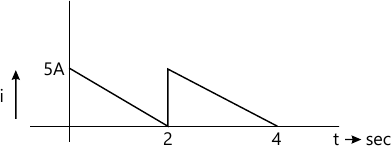MORE IN Basic Electricals & Electronics Engg.
RGPV First Year Engineering (Set B) (Semester 2)
Basic Electricals & Electronics Engg.
May 2013
Total marks: --
Total time: --
INSTRUCTIONS
(1) Assume appropriate data and state your reasons
(2) Marks are given to the right of every question
(3) Draw neat diagrams wherever necessary

Distinguish the following
1 (a) (i) 3 phase balanced and unbalanced supply with phasor diagram
3 M
Answer any one question from Q1 & Q2
1 (a) (ii) Voltage source and current source
4 M
1 (b) (i) Obtain current I in the given R-L-C parallel circuit under resonant condition. Justify your answer.3 M
1 (b) (ii) Obtain resultant voltage when two source of emfs having e1=100 sin ?t and $e_2=100 \sin \left( \omega - \dfrac {\pi}{6} \right )$ are connected in series. If resultant voltage is applied to circuit of impedance (8+j3)?, calculate the power (active) supplied to the impedance.
4 M

2 (a) Find the average and RMS value of the following waveforms. Also calculate form factor and peak factor of the same.7 M
2 (b) Explain Thevenin?s & superposition theorem giving an application example for each.
7 M

Answer any one question from Q3 & Q4
3 (a) Explain basic principle of operation of a transformer. Draw an equivalent circuit of single phase transformer.
7 M
3 (b) A single phase transformer rated 570 watt has an efficiency of 95 percent when working at full load and half full load, both at unity PF. Calculate its efficiency at 75 percent of full load.
7 M

Specify the following w.r.t. transformer.
4 (a) (i) All day efficiency
3 M
4 (a) (ii) Losses in the transformer
4 M
4 (b) How will you determine the transformer losses in the laboratory.
7 M

Answer any one question from Q5 & Q6
5 (a) Draw Torque-slip characteristics of three phase induction motor and explain its stable and unstable region of operation
7 M
Answer the following w.r.t. induction motor
5 (b) (i) What is the frequency of rotor currents of an induction motor?
3 M
5 (b) (ii) Why is an induction motor called asynchronous?
2 M
5 (b) (iii) What do you mean by space phase difference.
2 M

6 (a) State the types of dc motors. Discuss constructional details of any type of dc motor.
7 M
6 (b) A shunt generator delivers 50kw at 250V and 400r.p.m. The armature and field resistances are 0.027?and 50? respectively. Calculate the speed of the machine running as shunt motor and taking 50kw input at 250V.
7 M

7 (a) What are logic gates. Enlist the different types of logic gates.
4 M
7 (b) What is an EX-NOR Gate? Write its truth table.
3 M
7 (c) Verify that the following operations are commutative and associative.
i) AND ii) OR iii) EXOR
4 M
7 (d) Implement the following logic expressions with logic gates:
Y=ABC + AB + BC
Y=ABC (D + EF)
3 M
7 (e) Design a full adder circuit using NAND gates.
4 M
7 (f) State and explain De Morgan's theorem.
3 M
7 (g) How will you convert decimal number in octal.
4 M

8 (a) Draw Input/Output characteristic of a transistor in CE configuration.
4 M
8 (b) Discuss DC biasing of BJT
3 M
8 (c) How BJT can be used a
i) Switch ii) Inverter
4 M
8 (d) Discuss V-I characteristic of P.N Diode.
3 M
8 (e) Which transistor configuration CC, CB & CE is suitable for Amplifier and why.
4 M
8 (f) Differential between intrinsic & extrinsic semiconductor.
3 M

More question papers from Basic Electricals & Electronics Engg.